# Sets, Relations and Functions   Share

## What is Sets, Relations and Functions

In Mathematics, Topic sets, relation, and function is one of the very important topics as this topic serves as a basic building for whole calculus (differential and integral). Every year you will get at max 1 - 2 questions in JEE Main and other exams, directly (as chapter weight in jee main is only 5%) but indirectly, the concept of this chapter will be involved in whole calculus, differential and integral both part and function will be used in many other chapters. Sets, relations, and functions are building blocks for calculus and hence a very important chapter in the preparation of competitive entrance exams. Some students find Sets, relation and function little challenging to understand and solve the problems initially. But as you solve more and more problems on the Sets, relation and function you will get familiar with this topic. Afterward, the questions will appear easy for you. You will find sets little easy compared to relation and function, the concept of sets will be easy to visualize as you can understand them with the help of diagram (Venn diagram). All three topics of this Sets, relation and function are interrelated and earlier concepts of the chapter will be pre-requisite for the latter concept, like sets for relation and relation for function. Overall this chapter will be a little longer than other chapters.

## Why Sets, Relations, and Functions:

Sets, Relations, and functions find a wide range of application in real-life problem, for example

Making a playlist of your favorite song, in doing that you make a folder and add all your favorite song in that folder and if you name that folder as fav. Song then fav. The song is a set that contains your favorite songs. Sets are also used in programming data science for grouping the data.

Prepare Online for JEE Main/NEET

Crack JEE 2021 with JEE/NEET Online Preparation Program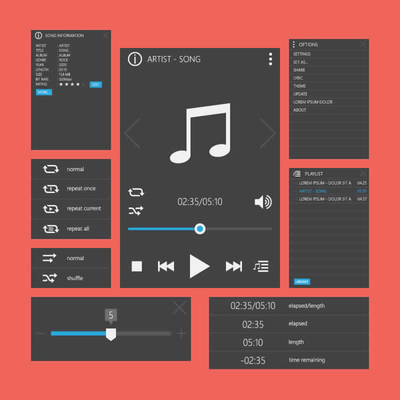Consider the relation of temperature and time at a particular place, with time, temperature keeps increasing till it reaches a certain maximum at some definite time and then it starts decreasing again, So we see that time is directly related to temperature, at any given time we get unique temperature, so this is a real-life use case of relations.

For function, we can think about it as a machine which gives you some definite and unique output for the input given by you, Lets see the example of driving a bike, if you are driving a bike you can’t be at two places at a given time, so your position while driving a bike will be a function of time, time being the input variable and your location as output variable.

Exponential decay of radioactive material is also an example of functions

## After studying Sets, Relations, and Functions :

1. After studying this chapter you will be comfortable with function and ready to dive in differential and integral calculus.

2. You will be able to solve questions related to Sets, Relations and Functions and the questions which require the use of all three concepts at the same time.

3. Since Sets, Relations, and functions are building blocks for calculus and a basic concept for many other chapters, so it will be helping you understand many other chapters easily.

4. And obviously, the chapter itself will help you to score some marks in the exam as it gets about 5% weight in jee main and around similar weight in other exams.

## Important topics :

1. Sets, roster and set builder form of sets

2. Type of sets, subset, the proper and improper subset

3. Power set, universal set, the union of sets, complement of sets

4. De-morgan’s law

5. Ordered pairs, cartesian product

6. Relation, number of relation, types of relation

7. Functions, image, and Pre-image

8. Types of functions

9. Composition of function, condition for composite function, the property of a composite function

10.  The inverse of a function

## Overview of the Sets, Relations, and Functions:

Set: a well-defined collection of distinct objects is called set, denoted by capital letters like A, Q, R, N, …

Type of sets: Empty set, equal set, equivalence set. Sets are said to be empty if they contain no element. Sets are said to be equal if they contain the same elements and number elements are also the same.

Subsets: a set A is said to be a subset of a set B if all elements of A present in B. And If the number of elements in A is not equal to the number of elements in B, then A is said to be a proper subset of B otherwise improper subset of B

Power set, universal set: The collection of all subsets of a set A is called the power set of A. A set that contains all sets in a given context is called the “Universal Set”. The universal set is usually denoted by U, and all its subsets denoted by the letters A, B, C, etc.

Relation and number of relation: A relation R from a non-empty set A to a non-empty set B is a subset of the cartesian product A × B. The subset is derived by describing the relationship between the first element and the second element of the ordered pairs in A × B.

If A has m elements and B has n elements, then AB has m x n element.  And the number of different relation from A to B isFunctions: A relation from a set A to a set B is said to be a function from A to B if every element of set A has one and only one image in set B.

Types of function: There are many types of function and they are classified depending upon their properties, behavior, range, etc. The function which is not algebraic is called transcendental function, like exponential functions, logarithmic function and so on. Other types of function are modulus function, piecewise functions, greatest integer function, trigonometric function. We will study all of these functions in good detail.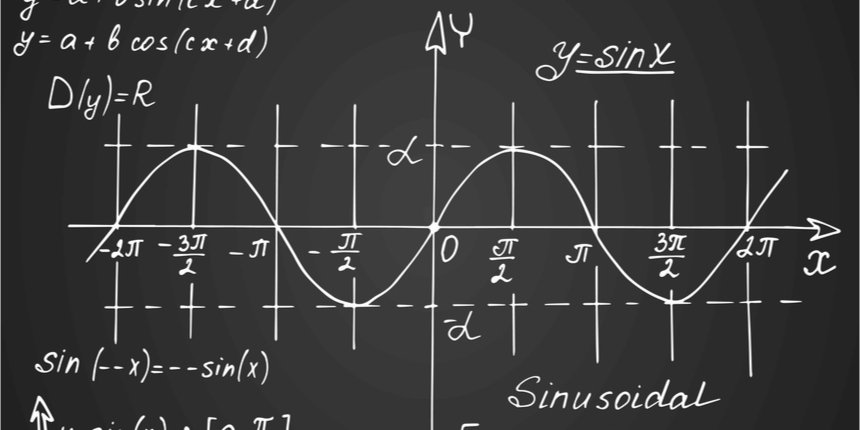Composition of function: Let f: AB and g: BC be two functions. Then the composition of f and g is denoted by gof and defined as the function gof: AC given by gof (x) = g(f (x)),xA.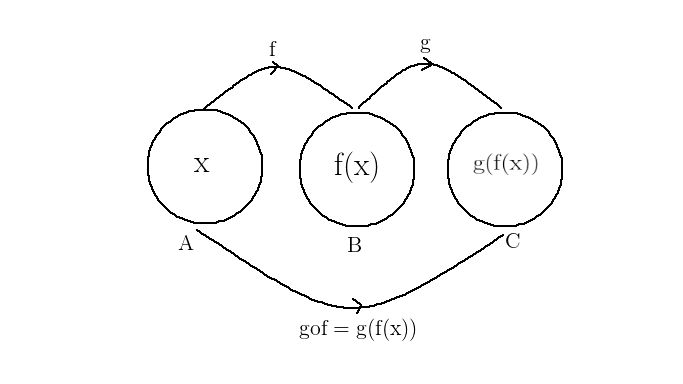Here Domain of gof = Domain of f and

Co-domain of gof = Co-Domain of g

The inverse of a function: Function f? X → Y is invertible if and only if f is a bijective function. If function f: X → Y is invertible, then there exists a function g: Y → X such thatand. The function g is called the inverse of f and is denoted by f -1 .

## How to prepare Sets, Relations, and Functions:

Sets, Relations, and Functions is a basic topic or you can say building block for differential and integral calculus and it is used in many other chapters also, so you must be through with this chapter.

1. To understand this topic you have to go in the order of the name of this chapter means first you must study sets then relation and after that function in last, then it will be easy to comprehend.

2.  Start with understanding basic concepts like simple definitions (what is set, what is a relation, what is a function), under each topic independently before moving to another by going through every concept.

3.  Once you’re clear with basic concepts, move to complex concepts, like properties of union and intersection, De-Morgan’s Laws, number of relation and function, and so on. In the end, you must be able to integrate all the topics to solve on the question.

4. After studying these concepts go through solved examples and then go to mcq and practice the problem to make sure you understood the topic.

5.  Solve the questions of the books which you are following and then go to previous year papers.

6. While going through concept make sure you understand the derivation of formulas and try to derive them by your own, as many times you will not need the exact formula but some steps of derivation will be very helpful to solve the problem if you understand the derivation it will boost your speed in problem-solving.

7. Since Sets, Relations, and Functions can be geometrically visualized try to understand and relate the things with Venn diagrams and graphs, as visualization of the problem makes the problem easy to comprehend and hence it becomes easy for us to solve it.

8. At the end of chapter try to make your own short notes for quick revision, make a list of formula to revise quickly before exams or anytime when you required to revise the chapter, it will save lots of time for you.

## Best books for the preparation of this Sets, Relations and Functions:

First, finish all the concept, example and questions given in NCERT Maths Book. You must be thorough with the theory of NCERT. Then you can refer to the book Algebra Arihant by Dr. SK goyal or RD Sharma or Cengage Mathematics Algebra but make sure you follow any one of these not all. Sets, Relations, and Functions are explained very well in these books and there are an ample amount of questions with crystal clear concepts. Choice of reference book depends on person to person, find the book that best suits you the best, depending on how well you are clear with the concepts and the difficulty of the questions you require.

## Maths Chapter-wise Notes for Engineering exams

 Chapters Chapters Name Chapter 2 Complex Numbers and Quadratic Equations Chapter 3 Matrices and Determinants Chapter 4 Permutations and Combinations Chapter 5 Binomial Theorem and its Simple Applications Chapter 6 Sequence and Series Chapter 7 Limit, Continuity, and Differentiability Chapter 8 Integral Calculus Chapter 9 Differential Equations Chapter 10 Coordinate Geometry Chapter 11 Three Dimensional Geometry Chapter 12 Vector Algebra Chapter 13 Statistics and Probability Chapter 14 Trigonometry Chapter 15 Mathematical Reasoning Chapter 16 Mathematical Induction

### Topics from Sets, Relations and Functions

• Sets and their represntation ( JEE Main, KCET, SRMJEEE, MET, MHT-CET, KEAM ) (52 concepts)
• Union ( JEE Main, KCET, SRMJEEE, MET, MHT-CET, KEAM ) (24 concepts)
• Intersection and complement of sets and their algebric properties ( JEE Main, KCET, SRMJEEE, MET, MHT-CET, KEAM ) (84 concepts)
• Power set ( JEE Main, KCET, SRMJEEE, MET, MHT-CET, KEAM ) (12 concepts)
• Relation ( JEE Main, KCET, SRMJEEE, MET, MHT-CET, KEAM ) (84 concepts)
• Types of relations ( JEE Main, KCET, SRMJEEE, MET, MHT-CET, KEAM ) (40 concepts)
• Functions ( JEE Main, KCET, SRMJEEE, MET, MHT-CET, KEAM ) (52 concepts)
• One-one , into and onto functions ( JEE Main, KCET, SRMJEEE, MET, MHT-CET, KEAM ) (44 concepts)
• Composition of functions ( JEE Main, KCET, SRMJEEE, MET, MHT-CET, KEAM ) (68 concepts)
• Trigonometrical identities and equations ( AEEE, JEE Main, TS EAMCET, AP EAMCET, COMEDK UGET ) (201 concepts)
• Trigonometrical functions, Inverse trigonometrical functions and their properties ( AEEE, JEE Main, TS EAMCET, AP EAMCET, COMEDK UGET ) (90 concepts)
• Height and distance ( AEEE, JEE Main, TS EAMCET, AP EAMCET, COMEDK UGET ) (12 concepts)
• Sets, Representation of Sets and Different Types of Sets ( JEE Main, KCET, SRMJEEE, MET, MHT-CET, KEAM ) (56 concepts)
• Cartesian Product, Relations and Types of Relations ( JEE Main, KCET, SRMJEEE, MET, MHT-CET, KEAM ) (35 concepts)
• Functions, Domain-range and Types of functions ( JEE Main, KCET, SRMJEEE, MET, MHT-CET, KEAM ) (90 concepts)
• Transformation of Graphs ( JEE Main, KCET, SRMJEEE, MET, MHT-CET, KEAM ) (27 concepts)
• Trigonometric Functions ( AEEE, JEE Main, TS EAMCET, AP EAMCET, COMEDK UGET ) (18 concepts)
• Trigonometric Ratios and Transformation Formulas ( AEEE, JEE Main, TS EAMCET, AP EAMCET, COMEDK UGET ) (26 concepts)
• Trigonometric Equations ( AEEE, JEE Main, TS EAMCET, AP EAMCET, COMEDK UGET ) (14 concepts)
• Properties and Solutions of Triangle ( AEEE, JEE Main, TS EAMCET, AP EAMCET, COMEDK UGET ) (28 concepts)
• Inverse Trigonometric Functions ( AEEE, JEE Main, TS EAMCET, AP EAMCET, COMEDK UGET ) (38 concepts)
• Principle of Mathematical Induction ( JEE Main, COMEDK UGET ) (2 concepts)
• Introduction ( JEE Main, KCET, MET, KVPY SA, KVPY SB/SX, COMEDK UGET ) (1 concepts)
• Logic Connectivity ( JEE Main, KCET, MET, KVPY SA, KVPY SB/SX, COMEDK UGET ) (1 concepts)
• Truth Table ( JEE Main, KCET, MET, KVPY SA, KVPY SB/SX, COMEDK UGET ) (1 concepts)
• Set Theoretical Approach of Logic Connectives ( JEE Main, KCET, MET, KVPY SA, KVPY SB/SX, COMEDK UGET ) (3 concepts)
• Practice Session ( JEE Main, KCET, MET, KVPY SA, KVPY SB/SX, COMEDK UGET ) (4 concepts)

### Important Books for Sets, Relations and Functions

•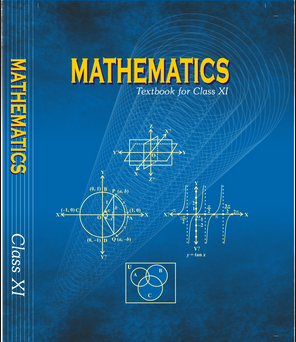•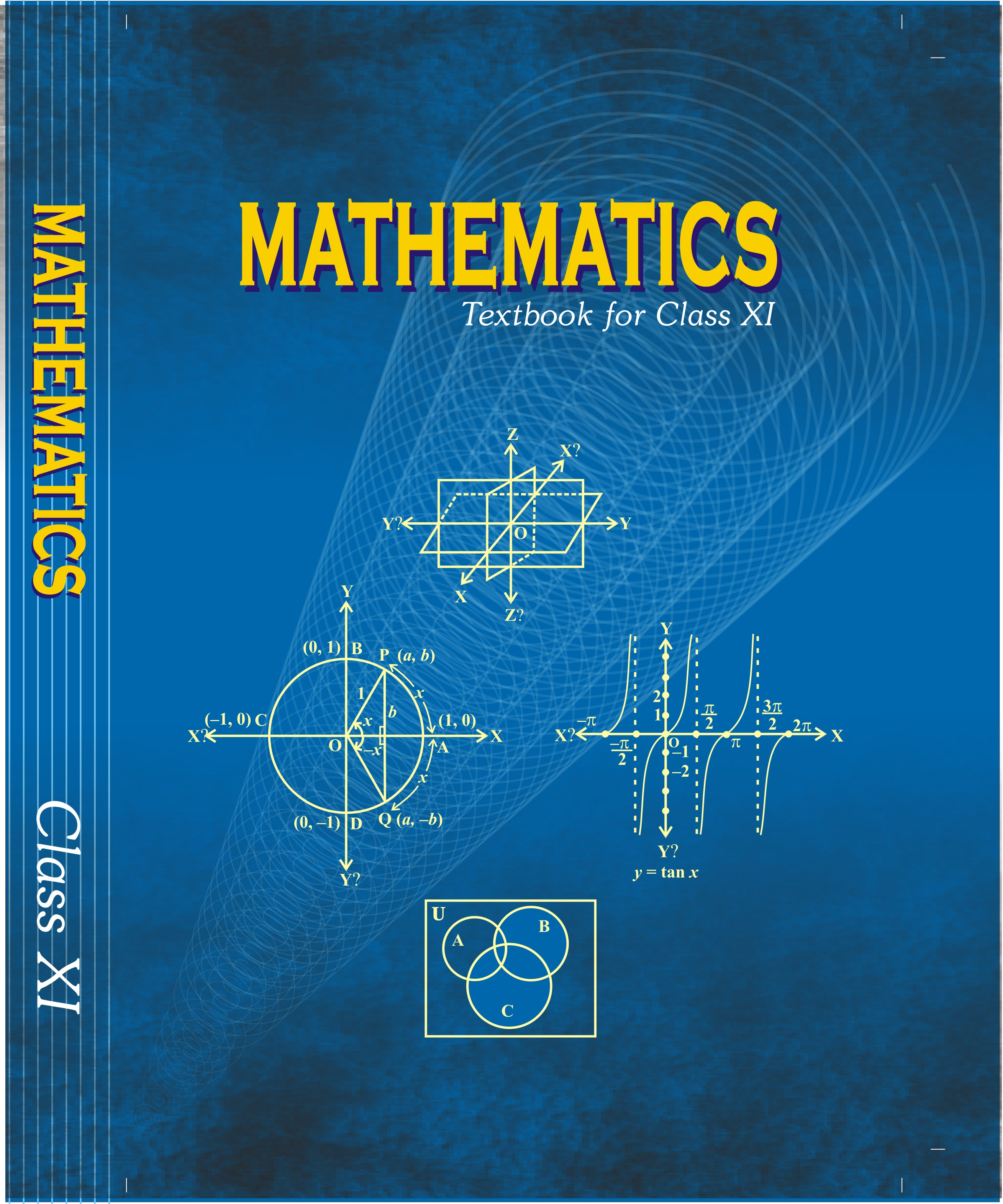•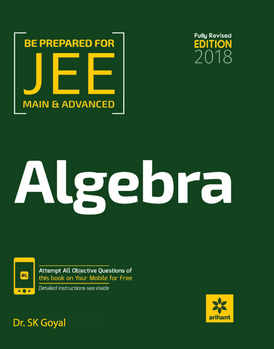Exams
Articles
Questions